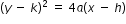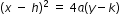# Cluster 1: Translate between the geometric description and the equation for a conic section. (Geometry - Additional Cluster) (Algebra 2 - Additional Cluster)Archived

Clusters should not be sorted from Major to Supporting and then taught in that order. To do so would strip the coherence of the mathematical ideas and miss the opportunity to enhance the major work of the grade with the supporting clusters.

General Information
Number: MAFS.912.G-GPE.1
Title: Translate between the geometric description and the equation for a conic section. (Geometry - Additional Cluster) (Algebra 2 - Additional Cluster)
Type: Cluster
Subject: Mathematics - Archived
Domain-Subdomain: Geometry: Expressing Geometric Properties with Equations

## Related Standards

This cluster includes the following benchmarks.

## Related Access Points

This cluster includes the following access points.

## Access Points

MAFS.912.G-GPE.1.AP.1a
Given the center and the radius of a circle, use the Pythagorean theorem to find the equation of the circle.
MAFS.912.G-GPE.1.AP.1b
Given the equation, find the center and the radius of a circle.
MAFS.912.G-GPE.1.AP.2a
Use the formulaor formulafor a parabola to write the equation when given the focus and directrix.

## Related Resources

Vetted resources educators can use to teach the concepts and skills in this topic.

## Formative Assessments

Complete the Square for Center-Radius 2:

Students are asked to find the center and radius of a circle given its equation in general form.

Type: Formative Assessment

Derive the Circle – General Points:

Students are given the coordinates of the center, (h, k), and the radius, r, of a circle and are asked to derive the equation of the circle using the Pythagorean Theorem.

Type: Formative Assessment

Derive the Circle – Specific Points:

Students are given the coordinates of the center and the radius of a circle and are asked to derive the equation of the circle using the Pythagorean Theorem.

Type: Formative Assessment

Students are asked to find the center and radius of a circle given its equation in general form.

Type: Formative Assessment

## Lesson Plans

I'm Focused on the Right Directrix:

In this lesson, the geometric definition of a parabola is introduced. Then students learn how to write the equation of a parabola in vertex form given its focus and directrix.

Type: Lesson Plan

Discovering Properties of Parabolas by Comparing and Contrasting Parabolic Equations:

• Teachers can use this resource to teach students how to derive the equation of a parabola in vertex form y = a(x – h)2 + k, when given the (x, y) coordinates of the focus and the linear equation of the directrix.
• An additional interactive graphing spreadsheet can be used as a resource to aid teachers in providing examples.

Type: Lesson Plan

Acting Out A Parabola: the importance of a vertex and directrix:

Students will learn the significance of a parabola's vertex and directrix. They will learn the meaning of what exactly a parabola is by physically representing a parabola, vertex, and directrix. Students will be able to write an equation of a parabola given only a vertex and directrix.

Type: Lesson Plan

Explore the Properties of a Parabola and Practice Writing its Equation:

This lesson provides an opportunity for students to:

• confirm the definition of a parabola by measuring the distances from parabola points to its focus and the directrix;
• discover parabolic parameters by measuring the distances from its focus to its vertex ("p"), its vertex to the directrix ("p"), and the length of its latus rectum ("4p");
• test the vertex-directrix form of parabola equation by choosing random values of the directrix' length and the coordinates of the vertex, using those values in writing vertex-directrix form of parabolic equations, and then by using graphing calculators identifying the type of curves described by those equations.

As a result of this lesson, students will learn the properties of parabola, be able to write an equation of a parabola given a focus and directrix, and will demonstrate these skills in completing the Independent Practice worksheet.

Type: Lesson Plan

Definition of a Parabola:

Student will learn how to determine an algebraic representation of a parabola, given its focus and its directrix.

Type: Lesson Plan

Ellipse Elements and Equations:

Students use both graphic and analytic methods to write the equation of an ellipse given foci and directrices.

Type: Lesson Plan

Anatomy of a Parabola:

Graphic and analytic means are used to write the equation of a parabola given its focus and directrix.

Type: Lesson Plan

Circle Reasoning:

Students use the Pythagorean Theorem (Distance Formula) to derive the Standard Equation of a Circle; then move between descriptions and equations of a circle.

Type: Lesson Plan

Equations of Circles 1:

This lesson unit is intended to help you assess how well students are able to use the Pythagorean theorem to derive the equation of a circle and translate between the geometric features of circles and their equations.

Type: Lesson Plan

Equations of Circles 2:

This lesson unit is intended to help you assess how well students are able to translate between the equations of circles and their geometric features and sketch a circle from its equation.

Type: Lesson Plan

The Math Behind the Records:

Students will develop an understanding of how the position of the focus and directrix affect the shape of a parabola. They will also learn how to write the equation of a parabola given the focus and directrix. Ultimately this will lead to students being able to write an equation to model the parabolic path an athlete's center of mass follows during the high jump.

Type: Lesson Plan

Run Fido, Run!:

A guided practice for deriving the equation of a circle and then identifying a location to tether a dog to maximize movement.

Type: Lesson Plan

A Point and a Line to a Parabola!:

In this lesson, the student will use the definition of a parabola and a graphing grid (rectangular with circular grid imposed) to determine the graph of the parabola when given the directrix and focus. From this investigation, and using the standard form of the parabola, students will determine the equation of the parabola.

Type: Lesson Plan

Introduction to the Conic Section Parabola:

This lesson is an introduction into conic sections using Styrofoam cups and then taking a closer look at the parabola by using patty paper to show students how a parabola is formed by a focus and a directrix.

Type: Lesson Plan

## Original Student Tutorials

Where IS that Cell Tower?:

Find the location and coverage area of cell towers to determine the center and radius of a circle given its equation, using a strategy completing the square in this interactive tutorial.

Type: Original Student Tutorial

Circle Up!:

Learn how to write the equation of a circle using Pythagorean Theorem given its center and radius using step-by-step instructions in this interactive tutorial.

Type: Original Student Tutorial

## Perspectives Video: Expert

Sonification and Eccentricity of the Sun:

You may know that you need to use your brain to do science, but did you know you need your ears, too?

Type: Perspectives Video: Expert

## Perspectives Video: Teaching Idea

Representations of Parabolic Functions:

Don't get bent out of shape! Here's some ideas about how parabolic functions are connected to the real world and different ways they can be represented.

Type: Perspectives Video: Teaching Idea

Slopes and Circles:

The purpose of this task is to lead students through an algebraic approach to a well-known result from classical geometry, namely, that a point X is on the circle of diameter AB whenever ?AXB is a right angle

## Virtual Manipulative

Cool Tool For Ellipses:

Use this interactive GeoGebraTube tool to see how the foci and other graph characteristics are related to the equation of the ellipse.  Make sure you use the sliders to change the characteristics of your ellipse and pay attention to how the graph relates to its equation each time.

Type: Virtual Manipulative

## Worksheet

Reflection by a Parabola:

Students will use the construction of copying an angle to implement the reflection of rays off a parabola. The students will observe that the reflected rays all pass through a common point, the focus.

Type: Worksheet

## Student Resources

Vetted resources students can use to learn the concepts and skills in this topic.

## Original Student Tutorials

Where IS that Cell Tower?:

Find the location and coverage area of cell towers to determine the center and radius of a circle given its equation, using a strategy completing the square in this interactive tutorial.

Type: Original Student Tutorial

Circle Up!:

Learn how to write the equation of a circle using Pythagorean Theorem given its center and radius using step-by-step instructions in this interactive tutorial.

Type: Original Student Tutorial

Slopes and Circles:

The purpose of this task is to lead students through an algebraic approach to a well-known result from classical geometry, namely, that a point X is on the circle of diameter AB whenever ?AXB is a right angle

## Virtual Manipulative

Cool Tool For Ellipses:

Use this interactive GeoGebraTube tool to see how the foci and other graph characteristics are related to the equation of the ellipse.  Make sure you use the sliders to change the characteristics of your ellipse and pay attention to how the graph relates to its equation each time.

Type: Virtual Manipulative

## Parent Resources

Vetted resources caregivers can use to help students learn the concepts and skills in this topic.